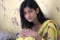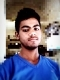## Knuth-Morris-Pratt AlgorithmUpdated on 15-Jun-2020 18:49:35
Knuth Morris Pratt (KMP) is an algorithm, which checks the characters from left to right. When a pattern has a sub-pattern appears more than one in the sub-pattern, it uses that property to improve the time complexity, also for in the worst case.The time complexity of KMP is O(n).Input and OutputInput: Main String: “AAAABAAAAABBBAAAAB”, The pattern “AAAB” Output: Pattern found at location: 1 Pattern found at location: 7 Pattern found at location: 14AlgorithmfindPrefix(pattern, m, prefArray)Input − The pattern, the length of pattern and an array to store prefix locationOutput − The array to store where prefixes are locatedBegin    length ... Read More

## kasai’s AlgorithmUpdated on 15-Jun-2020 19:00:01
Kasai’s Algorithm is used to get the Longest Common Prefix (LCP) array from suffix array. At first suffix arrays are found. After that Kasai's algorithm takes the suffix array list to find LCP.For the LCP array, it takes O(m log n), where m is pattern length and n is the length of the text. The Kasai’s Algorithm, it takes O(n) for searching the pattern in the main string.Input and OutputInput: Main String: “banana” Output: Suffix Array : 5 3 1 0 4 2 Common Prefix Array : 1 3 0 0 2 0AlgorithmbuildSuffixArray(text)Input: The main stringOutput: The suffix array, built ... Read More

## Efficient Construction of Finite AutomataUpdated on 15-Jun-2020 17:31:15
By constructing Finite Automata, we can simply perform the pattern searching in texts. At first, we have to fill a 2D array to make the transition table of the finite automata. Once the table is created, the searching procedure is simple. By starting from the first state of the automaton, when we reach the final state, it means that the pattern is found in the string.For finite automata construction, the time complexity is O(M*K), M is the pattern length and the K is a number of different characters. The complexity of main pattern searching is O(n).Input and OutputInput: Main String: ... Read More

## Boyer Moore AlgorithmUpdated on 15-Jun-2020 17:41:15
It is another approach of Boyer Moore Algorithm. Sometimes it is called the Good Suffix Heuristic method. For this case, a preprocessing table is created as suffix table. In this procedure, the substring or pattern is searched from the last character of the pattern. When a substring of main string matches with a substring of the pattern, it moves to find other occurrences of the matched substring. It can also move to find a prefix of the pattern which is a suffix of main string. Otherwise, it moves the whole length of the pattern.Input and OutputInput: Main String: “ABAAABCDBBABCDDEBCABC”, Pattern: ... Read MoreUpdated on 15-Jun-2020 17:45:08
The bad character heuristic method is one of the approaches of Boyer Moore Algorithm. Another approach is Good Suffix Heuristic. In this method we will try to find a bad character, that means a character of the main string, which is not matching with the pattern. When the mismatch has occurred, we will shift the entire pattern until the mismatch becomes a match, otherwise, pattern moves past the bad character.Here the time complexity is O(m/n) for best case and O(mn)for the worst case, where n is the length of the text and m is the length of the pattern.Input and ... Read More

## Anagram Pattern SearchUpdated on 15-Jun-2020 17:47:44
Anagrams are basically all permutations of a given string or pattern. This pattern searching algorithm is slightly different. In this case, not only the exact pattern is searched, it searches all possible arrangements of the given pattern in the text.To solve this problem, we will divide the whole texts into several windows of length same as patterns. Then count on each character of the pattern is found and stored in an array. For each window, we also try to find the count array, then check whether they are matching or not.The time Complexity of Anagram Pattern Search Algorithm is O(n).Input ... Read More

## Aho-Corasick AlgorithmUpdated on 15-Jun-2020 16:35:18
This algorithm is helpful to find all occurrences of all given set of keywords. It is a kind of Dictionary-matching algorithm. It uses a tree structure using all keywords. After making the tree, it tries to convert the tree as an automaton to make the searching in linear time. There are three different phases of Aho-Corasick Algorithm. These are Go-to, Failure, and Output. In the go-to stage, it makes the tree using all the keywords. In the next phase or in the Failure Phase, it tries to find the backward transition to get a proper suffix of some keywords. In the ... Read More

## Fractional Knapsack ProblemUpdated on 15-Jun-2020 17:07:11
A list of items is given, each item has its own value and weight. Items can be placed in a knapsack whose maximum weight limit is W. The problem is to find the weight that is less than or equal to W, and value is maximized.There are two types of Knapsack problem.0 – 1 KnapsackFractional KnapsackFor the 0 – 1 Knapsack, items cannot be divided into smaller pieces, and for fractional knapsack, items can be broken into smaller pieces.Here we will discuss the fractional knapsack problem.The time complexity of this algorithm is O(n Log n).Input and OutputInput: Maximum weight = ... Read More

## Prim’s MST for Adjacency List RepresentationUpdated on 15-Jun-2020 17:13:06
It is similar to the previous algorithm. Here the only difference is, the Graph G(V, E) is represented by an adjacency list.Time complexity adjacency list representation is O(E log V).Input and OutputInput: The cost matrix: Output: Edge: A--B And Cost: 1 Edge: B--E And Cost: 2 Edge: A--C And Cost: 3 Edge: A--D And Cost: 4 Edge: E--F And Cost: 2 Edge: F--G And Cost: 3 Total Cost: 15Algorithmprims(g: Graph, start)Input −  The graph g and the seed vertex named ‘start’Output − The Tree after adding edges.Begin    create two set B, N    add the start node in B ... Read More

## Prim’s Minimum Spanning Tree AlgorithmUpdated on 15-Jun-2020 17:22:04
There is a connected graph G(V, E) and the weight or cost for every edge is given. Prim’s Algorithm will find the minimum spanning tree from the graph G. It is a growing tree approach. This algorithm needs a seed value to start the tree. The seed vertex is grown to form the whole tree.The problem will be solved using two sets. One set holds the nodes that are already selected, and another set holds the item those are not considered yet. From the seed vertex, it takes adjacent vertices, based on minimum edge cost, thus it grows the tree by ... Read More